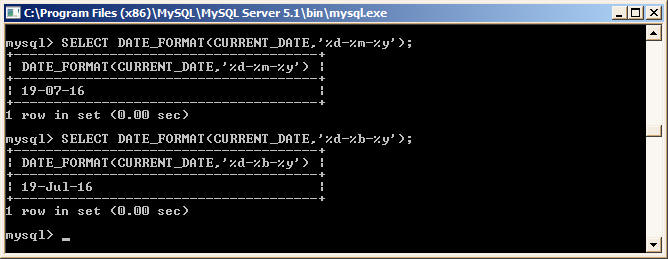﻿ FORMAT function in MSSQL and it's equivalent in MySQL# FORMAT Function in MS SQL Server and it's equivalent in MySQL

In MS SQL Serevr the FORMAT is used to format both Numeric Values to Strings and also Date and time values. Whereas in MySQL you have to use FORMAT function to format Numeric Values and DATE_FORMAT function to format Date & Time values.

Here are the details of these Functions

## MSSQL

The FORMAT function is introduced in MS SQL Server 2012 only.

FORMAT function Syntax in MSSQL

FORMAT ( value, format [, culture ] )

### Formatting Numeric Values

```SELECT FORMAT(123456789,'###-##-####') AS 'Custom Number Result';
----------------
123-45-6789

SELECT FORMAT(1.2365, 'N', 'en-us') AS 'Number Format'
,FORMAT(1.2365, 'G', 'en-us') AS 'General Format'
,FORMAT(1.2365, 'C', 'en-us') AS 'Currency Format'

Number Format General Format Currency Format
------------- -------------- ---------------
1.24 		1.2365		 \$1.24

```

### Formatting Dates

```SELECT FORMAT ( '02/08/2002', 'D', 'en-US' ) AS 'US English Result'
----------------
Friday, February 8, 2002

SELECT FORMAT ( '02/08/2002', 'D', 'en-gb' ) AS 'Great Britain English Result'
----------------
08 February 2002

```

## MySQL

In MySQL we can use FORMAT function to format numbers only and DATE_FORMAT function to format dates. Here are the examples

FORMAT Function

Syntax

`FORMAT(X,D[,locale])`

Formats the number `X` to a format like `'#,###,###.##'`, rounded to `D` decimal places, and returns the result as a string. If `D` is `0`, the result has no decimal point or fractional part.

```mysql> select format(123.4567,3);
+--------------------+
| format(123.4567,3) |
+--------------------+
| 123.457 |
+--------------------+
1 row in set (0.00 sec)

mysql> select format(123.4567,1);
+--------------------+
| format(123.4567,1) |
+--------------------+
| 123.5 |
+--------------------+
1 row in set (0.00 sec)

mysql> select format(123.4567,5);
+--------------------+
| format(123.4567,5) |
+--------------------+
| 123.45670 |
+--------------------+
1 row in set (0.00 sec)```
```mysql> select format(12345.6789,5);
+----------------------+
| format(12345.6789,5) |
+----------------------+
| 12,345.67890 |
+----------------------+
1 row in set (0.00 sec)```
```mysql> select format(12345.6789,0);
+----------------------+
| format(12345.6789,0) |
+----------------------+
| 12,346 |
+----------------------+
1 row in set (0.00 sec)

mysql>
```

### DATE_FORMAT

DATE_FORMAT function has to be used in MySQL to format dates. It provides lot of formatting symbols to convert dates into any format you like.

Here is the syntax of this function

`DATE_FORMAT(date,format)`

Formats the `date` value according to the `format` string.

Here are the date format symbols which you can use in DATE_FORMAT function to format the dates and times

Format Symbol Description
`%a` Abbreviated weekday name (`Sun`..`Sat`)
`%b` Abbreviated month name (`Jan`..`Dec`)
`%c` Month, numeric (`0`..`12`)
`%D` Day of the month with English suffix (`0th`, `1st`, `2nd`, `3rd`, …)
`%d` Day of the month, numeric (`00`..`31`)
`%e` Day of the month, numeric (`0`..`31`)
`%f` Microseconds (`000000`..`999999`)
`%H` Hour (`00`..`23`)
`%h` Hour (`01`..`12`)
`%I` Hour (`01`..`12`)
`%i` Minutes, numeric (`00`..`59`)
`%j` Day of year (`001`..`366`)
`%k` Hour (`0`..`23`)
`%l` Hour (`1`..`12`)
`%M` Month name (`January`..`December`)
`%m` Month, numeric (`00`..`12`)
`%p` `AM` or `PM`
`%r` Time, 12-hour (`hh:mm:ss` followed by `AM` or `PM`)
`%S` Seconds (`00`..`59`)
`%s` Seconds (`00`..`59`)
`%T` Time, 24-hour (`hh:mm:ss`)
`%U` Week (`00`..`53`), where Sunday is the first day of the week; `WEEK()` mode 0
`%u` Week (`00`..`53`), where Monday is the first day of the week; `WEEK()` mode 1
`%V` Week (`01`..`53`), where Sunday is the first day of the week; `WEEK()` mode 2; used with `%X`
`%v` Week (`01`..`53`), where Monday is the first day of the week; `WEEK()` mode 3; used with `%x`
`%W` Weekday name (`Sunday`..`Saturday`)
`%w` Day of the week (`0`=Sunday..`6`=Saturday)
`%X` Year for the week where Sunday is the first day of the week, numeric, four digits; used with `%V`
`%x` Year for the week, where Monday is the first day of the week, numeric, four digits; used with `%v`
`%Y` Year, numeric, four digits
`%y` Year, numeric (two digits)
`%%` A literal `%` character
`%x` `x`, for any `x` not listed above

### Examples

```mysql> SELECT DATE_FORMAT(CURRENT_DATE,'%W');
+--------------------------------+
| DATE_FORMAT(CURRENT_DATE,'%W') |
+--------------------------------+
| Tuesday |
+--------------------------------+
1 row in set (0.00 sec)

mysql> SELECT DATE_FORMAT(CURRENT_DATE,'%W %D %M %Y ');
+------------------------------------------+
| DATE_FORMAT(CURRENT_DATE,'%W %D %M %Y ') |
+------------------------------------------+
| Tuesday 19th July 2016 |
+------------------------------------------+
1 row in set (0.00 sec)

mysql> SELECT DATE_FORMAT(CURRENT_DATE,'%W, %D %M %Y ');
+-------------------------------------------+
| DATE_FORMAT(CURRENT_DATE,'%W, %D %M %Y ') |
+-------------------------------------------+
| Tuesday, 19th July 2016 |
+-------------------------------------------+
1 row in set (0.00 sec)
```
```mysql> SELECT DATE_FORMAT(CURRENT_DATE,'%d-%m-%y');
+--------------------------------------+
| DATE_FORMAT(CURRENT_DATE,'%d-%m-%y') |
+--------------------------------------+
| 19-07-16 |
+--------------------------------------+
1 row in set (0.00 sec)

mysql> SELECT DATE_FORMAT(CURRENT_DATE,'%d-%b-%y');
+--------------------------------------+
| DATE_FORMAT(CURRENT_DATE,'%d-%b-%y') |
+--------------------------------------+
| 19-Jul-16 |
+--------------------------------------+
1 row in set (0.00 sec)```
``

Back to Converting Functions from MSSQL to MySQL